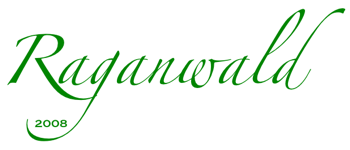(This is a snapshot of my old weblog. New posts and selected republished essays can be found at raganwald.com.)

Tuesday, February 06, 2007

In a comment on But Y would I want to do a thing like this?, Tom Moertel derived an elegant version of the actual Y combinator in Ruby. I reproduce his words here with permission.

Tom uses the alternate syntax for
`call`, `[...]`. I avoided this to keep clear of a tiresome debate about operator overloading. (On one side: those who hate it. On the other side: those that hate Ruby disallowing overloading `(...)`).

Ok Tom, take it away!

Here’s my original Ruby definition:

``def y(&f)    lambda { |*args| f[y(&f)][*args] }end``

To get rid of the recursive call `y(&f)`, let’s replace it with a variable `yf`, whose value we’ll say is magically equivalent to the result of the recursive call. To effect the binding of `yf`, we’ll need to wrap the method body in a new lambda expression and pass in the magic value:

``def y(&f)    lambda { |yf|        lambda { |*args| f[yf[yf]][*args] }    }[ <<< placeholder for yf: we'll fill this hole next >>> ]end``

So, what value should we pass in for `yf`? Because the variable represents the value that would have been computed by calling `y(&f)`, which is just the body of the `y` method, we can fill the hole with a duplicate of the body:

``def y(&f)    lambda { |yf|        lambda { |*args| f[yf[yf]][*args] }    }[ lambda { |yf| lambda { |*args| f[yf[yf]][*args] } } ]end``

And we have our Y combinator:

``fac = y { |rec| lambda { |n| n < 2 ? 1 : n * rec[n-1] } }fac # ==> 120``

While the combinator works, its implementation repeats itself: there are two copies of the lambda expression

``lambda { |yf| lambda { |*args| f[yf[yf]][*args] } }``

in the method body. To factor them out, let’s let the variable `x` represent this expression. Then the method body simplifies to `x[x]`, which is very satisfying. To set `x` to the correct value, however, we need to add a wrapper lambda that binds `x` to the original expression. Putting it all together, we arrive at the final version:

``def y(&f)    lambda { |x| x[x] } [         lambda { |yf| lambda { |*args| f[yf[yf]][*args] } } ]end``

And it, too, works:

``acc_fac = y { |rec| lambda { |n,acc| n < 2 ? acc : rec[n-1, n*acc] } }tail_fac = lambda { |n| acc_fac[n, 1] }tail_fac # ==> 120``

Cheers! —Tom

Labels: ,

Comments on “Guest Blogger: Tom Moertel derives the Y combinator in Ruby:
Here is another derivation of Y combinator in JavaScript: http://blog.csdn.net/g9yuayon/archive/2006/09/24/1271319.aspx.

It's written in Chinese, but the code speaks for itself. :-)

I think I finally understand this Y Combinator thing now. Hooray. So I explained it in my words.

<< Home